# VBA SolverArticle byExcelMojo Team## What is Excel VBA Solver?

VBA Solver is an add-in tool available to solve complex logical models where the user provides the inputs and the target result, and the VBA Solver shows the required output to achieve the specified target.

VBA Solver is a simulator model available to create engineering models, where it takes inputs from users with cell references as its parameters. For example, look at the following data in Excel.

In cell B5, we have applied the SUM excel formula, which takes cell references from cells B2 to B4. Assume we must get 189 in cell B5 by changing the value in cell B3 which is left blank. In these cases, VBA Solver helps us to solve this equation by running the simulator.

The following VBA Solver code will run the VBA Solver and get us the required value in cell B3 to make the value in cell B5 as 189. Once the code is run, VBA Solver inserts the value 88 in cell B3 to make the value in cell B5 as 189.

###### Key Takeaways
• VBA Solver solves complex problems and models to arrive at an optimum solution.
• To solve the equation, VBA Solver uses SolverOk, SolverAdd, and SolverSolve functions.
• We can apply the desired value in SolverOk and criteria in the SolverAdd function. After adding these two, we must apply the SolverSolve function. With the SolverSolve function, we can achieve the VBA Solver solution.

### How to Enable Solver in Worksheet?

Then, follow the steps below to allow the Solver in a worksheet. Follow the steps listed below to enable the Solver in a worksheet.

1. Go to the File tab.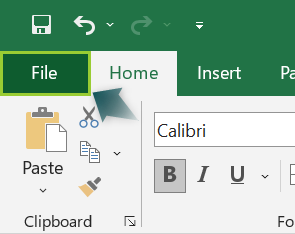2. Under the File tab, click “Options.”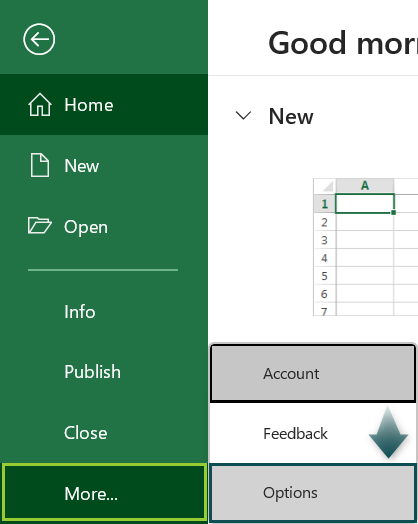Note: If you don’t see the “Options” under the File tab, click “More…”

3. Once you click “Options,” you will see the “Excel Options” window. Here, click on “Add-ins.”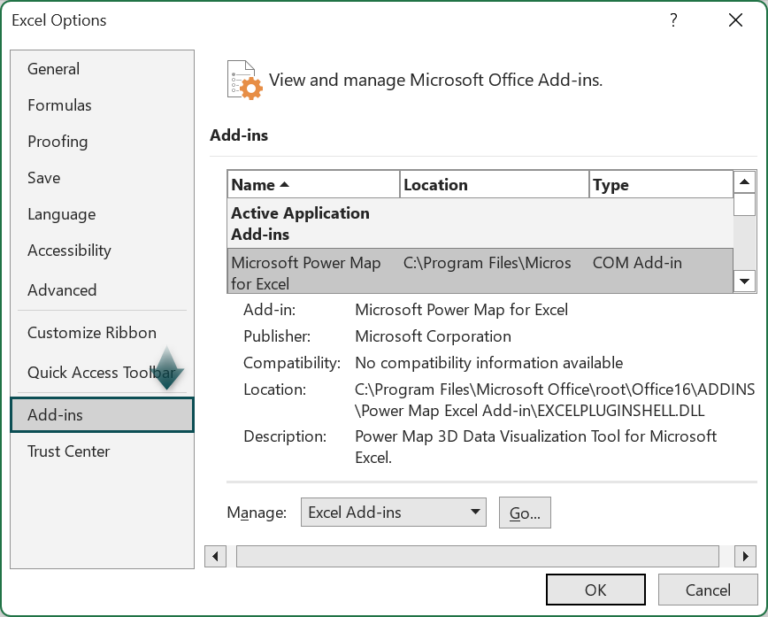4. Once “Add-ins” is selected, on the right-side screen, choose “Excel Add-ins” from the Manage drop-down list.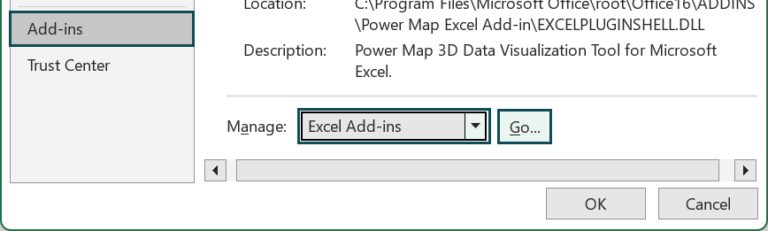5. Click “Go” to see the following “Add-ins” window.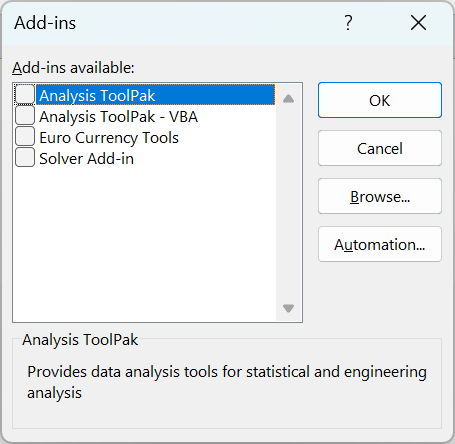As observed, “Solver Add-in” is not checked. Now, check the box.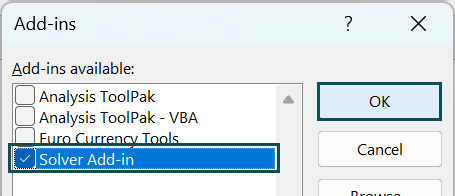6. Click “Ok.” Now we can see the “Solver Add-in” under the Data tab.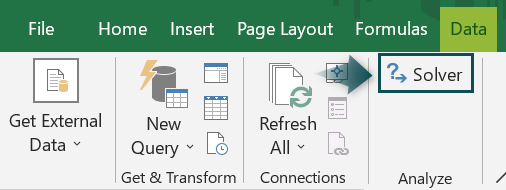### How to Enable Solver in VBA?

The VBA Solver is an external tool. Therefore, we need to set the object reference to it through the following steps.

• Step 1: Go to the Visual Basic Editor window by pressing the ALT + F11 shortcut key from the Excel worksheet.
• Step 2: Hover over the “Tools” tab and click “References”.
• Step 3: Now, we will see the References VBA project window. From this window, choose the “Solver” option.
• Step 4: Click OK, and now you should be able to use the “VBA Solver.”

### Solver Functions in VBA

Once the VBA Solver is enabled, we can see three different functions to use it. Following are the three other functions available under VBA solver.

1. SolverOk
3. SolverSolve
1. Syntax of the SolverOk function

SolverOk([SetCell], [MaxMinVal], [ValueOf], [ByChange], [Engine], [EngineDesc])

• SetCell: In this argument, we give the cell reference where we must obtain the eventual result.
• MaxMinVal: In this argument, we can use any of the following VBA Solver options.
• 1 = Maximize value of
• 2 = Minimize value of
• 3 = To the exact value of
• ValueOf: If we use the previous argument MaxMinVal then we must set this argument value. If we want the solver to give us the exact value of 100, then MaxMinVal will have a value of 3, and ValueOf will be 100.
• ByChange: In this argument, we must provide the cell which needs to be amended to get the desired value in SetCell.

The remaining two arguments not required and optional; hence, they can be ignored. -Once the SolverOk is written, we can write the SolverAdd function.

1. Syntax of the SolverAdd function

• CellRef: This cell reference is from the SolverOk function argument, ByChange. We must reference the same cell here to set the criteria for the changing cell.
• Relation: In this argument, we can set the limitations and conditions for the changing cell. For example, if we do not want the changing cell to not go beyond 100, then we can use the equal sign. The following are the integer numbers we can use to apply the condition.
• 1: Less than or equal to (<=)
• 3: Greater than or equal to (>=)
• 2: Equal to (=)
• 4: Must have final values that are integers
• 5: Must have values between 0 to 1
• 6: Must have final values that are all different and integers.

To understand the syntax better, we will show you a simple example to understand them practically.

### Example of Solver in Excel VBA

#### Example #1

For example, Peter is studying in Class 10 and has attended six exams out of 7. Following are the anticipated scores from six completed exams.

Now, let’s calculate the average score for these six subjects by applying the AVERAGE excel function in B9 Cell as follows.

As of now, the average score is 89 out of 6 subjects. However, Peter aims to achieve an average score of 90, and he would like to know his target score in the upcoming exam, “G,” to achieve this average. Let’s write a VBA Solver code to get the targeted score in the exam “G.”

• Step 1: Start a subroutine procedure by naming the VBA Solver macro.
• Step 2: Enter the SolverOk function.
• Step 3: The first argument of the SolverOk function is “SetCell,” i.e., the cell which needs to be affected by the Solver method, i.e., the average cell (B9).
• Step 4: Next, we must enter 3 for the MaxMinVal argument because we must make the Average cell value equal to 90.
• Step 5: Next, since we need to get the value of 90 for the ValueOf argument, we must enter the value as 90.
• Step 6: Finally, we must provide the cell range for the ByChange argument. For this argument, we must give the cell whose value needs to be changed to get the SetCell value. In this case, we must know what his marks in the final exam “G” should be to get the required average. Hence, give the cell reference as cell B8.

The remaining arguments of the SolverOk function are not required. Hence, they can be ignored. It concludes the SolverOk function.

• Step 7: Next, add the SolverAdd function to set the criteria, if any.
• Step 8: For the CellRef argument, give the cell reference B8 because it is the cell we must change to get the desired result.
• Step 9: Next, for the relation argument, enter the integer value two because we must set this to be equal to the value 90.
• Step 10: Now, to execute all the written codes, we must use the SolverSolve function at the end.

Code:

Sub Solver_Example1()
SolverOk SetCell:=Range(“B9”), MaxMinVal:=3, ValueOf:=90, ByChange:=Range(“B8”)
SolverSolve
End Sub

Let’s execute the code and we will get the following result.

Therefore, to get an overall average score of 90, Peter should score 94 marks in the final examination G.

#### Example #2 – Apply Criteria in VBA Solver

The real power of VBA Solver comes with criteria we can define as constraints to obtain the eventual result.

For example, assume you are a Mobile manufacturer, and you have a target of earning \$5000 as profit. You are not sure of how many units to produce and at what selling price you need sell.

Following is the information about the Mobile units.

The only information available here is the estimated cost per mobile. Therefore, we must find out how many units we have to sell and at what price to earn a profit of \$5,000.

The following conditions should be applied to get a profit of \$5,000.

• Total mobile units to be sold should not be more than 100.
• Price per mobile should not be more than \$90.

These two criteria we need to apply; the following code will give you an idea about that.

Code:

Sub Solver_Example2()
SolverOk SetCell:=Range(“B9”), MaxMinVal:=3, ValueOf:=5000, ByChange:=Range(“B2:B3”)
SolverSolve
End Sub

It will give us the following result when we run the code.

We must sell 99 mobiles at \$90 to earn an approximate profit of \$4950, which is closest to the targeted \$5000 profit.

### Important Things to Note

• VBA Solver is not enabled by default. First, we must enable it on the worksheet and then at the Visual Basic Editor level.
• VBA Solver works only in one sheet of a workbook.
• In VBA SolverOk function, 1 = Maximize value of, 2 = Minimize value of, 3 = To the exact value of
• In VBA SolverAdd, 1 = Less than or equal to (<=), 3 = Greater than or equal to (>=), 2 = Equal to (=).

1. What is an alternative for VBA Solver in Excel?

Sometimes, we can use VBA Goal Seek as an alternative to VBA Solver. However, not all the scenarios are best suited for VBA Goal Seek.

2. What is the Difference Between Goal Seek and Solver in Excel VBA?

Goal Seek helps us find the required value to achieve the desired result without constraints.
VBA Solver helps us find the required value to achieve the desired result with many criteria put in place.

3. Why is Excel VBA Solver Not Working?

First, we must enable the VBA solver in Excel; then, only we can use the VBA Solver. Therefore, if you have received the VBA Solver code from someone else, then first make sure you are enabling the VBA Solver before you use it.

4. Can Excel VBA Solver have two objectives?

Yes, it is possible to have two objective cells in the VBA solver; however, one must design the template so that the VBA solver runs smoothly.